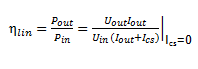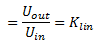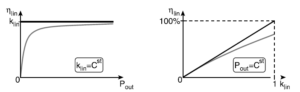# DC-DC Converter

Dc-Dc converters are employed in a variety of applications, such as :

1. Power supplies for personal computers
2. Office equipment
3. Spacecraft power systems
4. Laptop computers
5. Telecommunications equipment
6. Dc motor drives.

The input to a dc-dc converter is an unregulated dc voltage Vg and the output is  a regulated output voltage V, having a magnitude (and possibly polarity) that differs from Vg.

For example, in a computer off-line power supply, the 120 V or 240 V ac utility voltage is rectified, producing a dc voltage of approximately 170 V or 340 V, respectively. A dc-dc converter then reduces the voltage to the regulated 5 V or 3.3 V required by the processor ICs.

Several methods exist to achieve DC-DC voltage conversion. Each of these methods has its specific benefits and disadvantages, depending on a number of operating conditions and specifications.

Examples of such specifications are the voltage conversion ratio range, the maximal output power, power conversion efficiency, number of components, power density, galvanic separation of in- and output, etc.

## Linear Voltage Converters

The most elementary DC-DC converters are linear voltage converters.

They achieve DC-DC voltage conversion by dissipating the excess power into a resistor, making them resistive dividers. Clearly, this is not quite ideal for the power conversion efficiency .

Another implication of their operating principle is the fact that they can only convert a certain input voltage into a lower output voltage , having the same polarity. In other words, the value of their voltage conversion ratio is always between zero and one.

The advantage of linear voltage converters is that they are fairly simple to implement. Moreover, they generally do not need large, and space consuming inductors or capacitors, making them an attractive option for monolithic integration .

Therefore, the two types of linear voltage converters, namely the series and the shunt regulator, are discussed below .

## Series Converter

The operating principle of a linear series voltage converter is shown in Fig.1(a).Fig.1 : (a) The principle of a linear series voltage converter and (b) a simple practical implementation

A variable resistor Rseries is placed in series with the load RL, lowering the input voltage Uin to output voltage Uout.

The resistance of Rseries is controlled by the control system, which keeps Uout constant under varying values of Uin and RL, by measuring Uout.

The control system also consumes power, which is illustrated by its supply current Ics.

In this case the control system uses Uin as supply voltage, which can also be provided by Uout. However, the latter case will require a start-up circuit, as Uout is initially zero.

A practical implementation example for a linear series voltage converter is shown in Fig. 1(b).

In this example Rseries is implemented as an NPN BJT and the control system as an OPerational AMPlifier (OPAMP), which performs the task of an error amplifier.

By doing so, Uout is determined as follows :By examining the operating principle of Fig.1(a), the efficiency ηlin can be calculated as follows:When Ics is neglected and assumed to be zero, ηlin is equal to the voltage conversion ratio  klin and thus independent of RL. This is graphically illustrated by the black curve in Fig. 2(a). The gray curve illustrates the more realistic situation, where  Ics has a finite positive value. It can be seen that  ηlin will tend to decrease when Pout decreases .(a)                                                                            (b)

Fig.2

Fig.2 (a) shows the power conversion efficiency   ηlin as a function of the output power Pout for a linear
series voltage converter, at a constant voltage conversion ratio klin  .

The black curve is valid for a zero control system supply current Ics  and the gray curve is valid for a non-zero Ics

Fig.2 (b)  shows the power conversion efficiency ηlin as a function of the voltage conversion ratio klin for a linear series voltage converter, at a constant output power Pout.

The black curve is valid for a zero control system supply current Ics and the gray curve is valid for a non-zero Ics .

Clearly, linear series voltage converters have an intrinsic advantage, in terms of power conversion efficiency, at high voltage conversion ratios. This is illustrated by Fig. 2(b), where the black curve is valid for Ics = 0 and the gray curve for a finite, non-zero Ics. The gray curve shows that the impact of the power consumption of the control system on ηlin becomes more dominant when klin approaches unity.

This type of converter is well suited for monolithic integration, due to its simple nature and lack of large passives. However, as the excess power is dissipated in Rseries, the maximal Pout is limited by the allowed on-chip power dissipation. This limitation becomes more dominant for low values of klin.

This type of voltage converter are used in several designs in the work for start-up and rail-shifting .